# CV for NBA clutch free throws models

### Chapter 5.1: Cross-validation

The NBA clutch free throws data set has three variables for player $$i=1,…,10$$:

1. $$Y_i$$ is the number clutch free throws made
2. $$N_i$$ is the number clutch free throws attempted
3. $$q_i$$ is the proportion of the non-clutch free throws made

We model these data as $Y_i\sim\mbox{Binomial}(N_i,p_i),$ where $$p_i$$ is the true probability of making a clutch shot. The objective is to explore the relationship between clutch and overall percentages, $$p_i$$ and $$q_i$$. We do this using two logistic regression models:

1. $$\mbox{logit}(p_i) = \beta_1 + \beta_2\mbox{logit}(q_i)$$
2. $$\mbox{logit}(p_i) = \beta_1 + \mbox{logit}(q_i)$$

In both models we select uninformative priors $$\beta_j\sim\mbox{Normal}(0,10^2)$$.

In the first model, $$p_i=q_i$$ if $$\beta_1=0$$ and $$\beta_2=1$$; in the second model $$p_i=q_i$$ if $$\beta_1=0$$. Therefore, we compare the posteriors of the $$\beta_j$$ to these values to analyze the relationship between $$p_i$$ and $$q_i$$.

Y <- c(64, 72, 55, 27, 75, 24, 28, 66, 40, 13)
N <- c(75, 95, 63, 39, 83, 26, 41, 82, 54, 16)
q <- c(0.845, 0.847, 0.880, 0.674, 0.909, 0.899, 0.770, 0.801, 0.802, 0.875)

X <- log(q)-log(1-q) # X = logit(q)


## Plot the data

inits   <- c("RW","JH","KL","LJ","SC","IT","GA","JW","AD","KD")
plot(100*q,100*Y/N,
xlim=100*c(0.65,0.95),ylim=100*c(0.65,0.95),
xlab="Overall percentage",ylab="Clutch percentage")
text(100*q,100*Y/N+1,inits)
abline(0,1)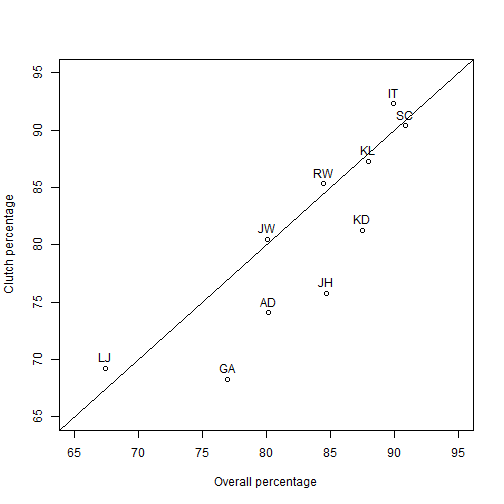expit <- function(x){1/(1+exp(-x))}


## Randomly assign observations to $$K=5$$ folds

 set.seed(0820)
fold <- rep(1:5,2)
fold <- sample(fold)
fold

##   3 1 5 2 1 2 4 5 4 3


## Fit the model and make predictions

## Define the models in JAGS

 library(rjags)

## Warning: package 'rjags' was built under R version 3.5.2

## Loading required package: coda

## Linked to JAGS 4.2.0

## Loaded modules: basemod,bugs

 Y_mean   <- matrix(NA,10,2)
Y_median <- matrix(NA,10,2)
Y_low    <- matrix(NA,10,2)
Y_high   <- matrix(NA,10,2)

for(f in 1:5){

# Select training data with fold not equal to f
data   <- list(Y=Y[fold!=f],N=N[fold!=f],X=X[fold!=f],n=sum(fold!=f))
params <- c("beta")

# Fit model 1
m1 <- textConnection("model{
for(i in 1:n){
Y[i]        ~ dbinom(p[i],N[i])
logit(p[i]) <- beta + beta*X[i]
}
beta ~ dnorm(0,0.01)
beta ~ dnorm(0,0.01)
}")
model1 <- jags.model(m1,data = data, n.chains=1,quiet=TRUE)
update(model1, 10000, progress.bar="none")
b1     <- coda.samples(model1, variable.names=params, thin=5, n.iter=20000, progress.bar="none")[]

# Fit model 2
m2 <- textConnection("model{
for(i in 1:n){
Y[i]        ~ dbinom(p[i],N[i])
logit(p[i]) <- beta + X[i]
}
beta ~ dnorm(0,0.01)
}")
model2 <- jags.model(m2,data = data, n.chains=1,quiet=TRUE)
update(model2, 10000, progress.bar="none")
b2     <- coda.samples(model2, variable.names=params, thin=5, n.iter=20000, progress.bar="none")[]

# Make predictions
for(i in 1:10){if(fold[i]==f){
Y_mod1        <- rbinom(nrow(b1),N[i],expit(b1[,1] + b1[,2]*X[i]))
Y_mean[i,1]   <- mean(Y_mod1)
Y_median[i,1] <- median(Y_mod1)
Y_low[i,1]    <- quantile(Y_mod1,0.025)
Y_high[i,1]   <- quantile(Y_mod1,0.975)

Y_mod2        <- rbinom(length(b2),N[i],expit(b2 + X[i]))
Y_mean[i,2]   <- mean(Y_mod2)
Y_median[i,2] <- median(Y_mod2)
Y_low[i,2]    <- quantile(Y_mod2,0.025)
Y_high[i,2]   <- quantile(Y_mod2,0.975)

ppd1 <- table(Y_mod1-0.1)
ppd2 <- table(Y_mod2+0.1) # Add 0.1 to avoid overlap

plot(ppd1,main=paste("Observation", i))
lines(ppd2,col=2)
abline(v=Y[i],lwd=2,col=3)

legend("topleft",c("PPD Model 1","PPD Model 2","Observed"),lwd=c(1,1,2),col=1:3,bty="n")
}}

rm(model1)
rm(model2)
}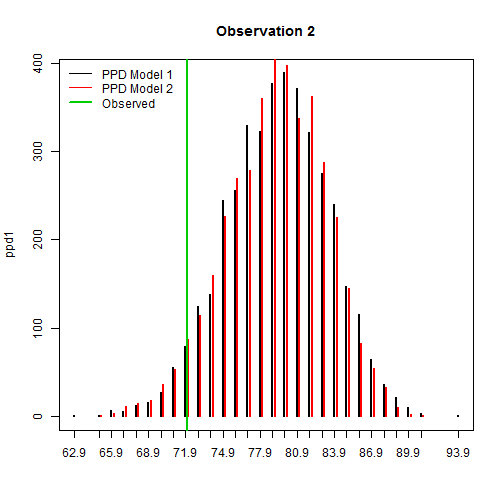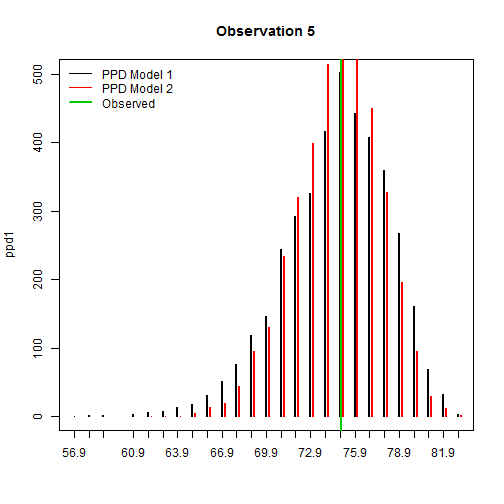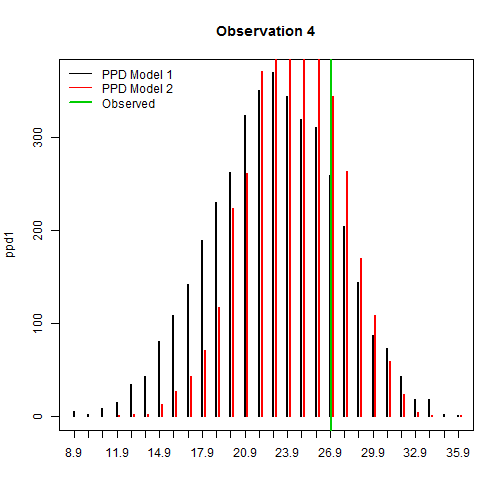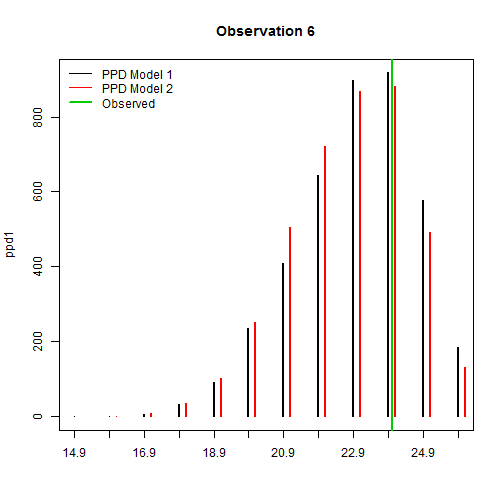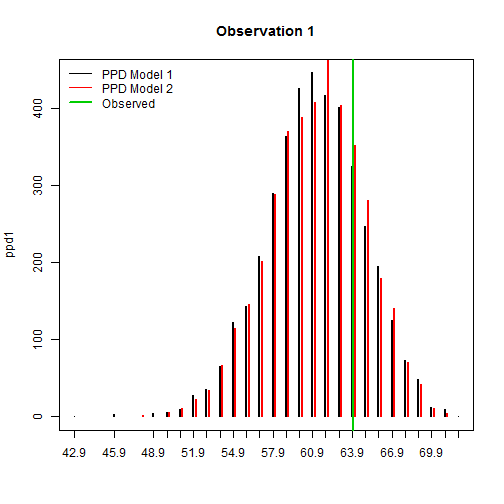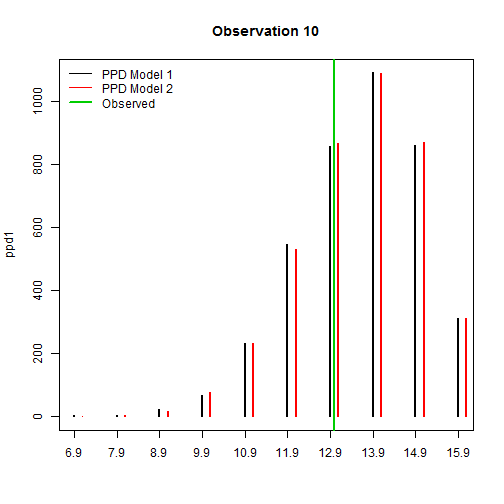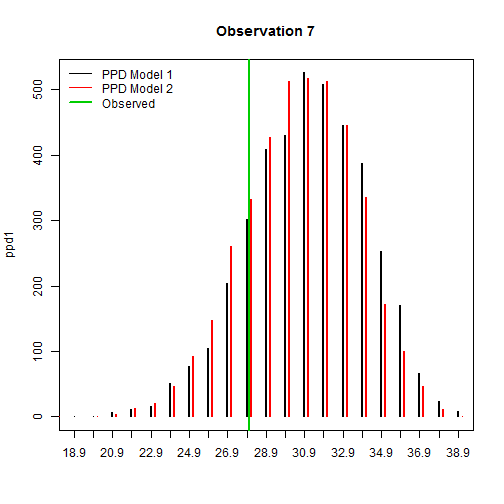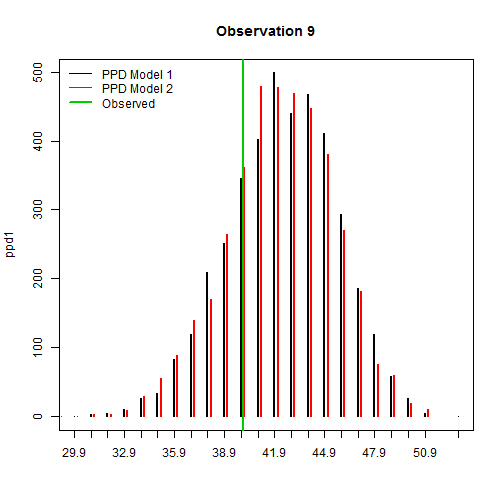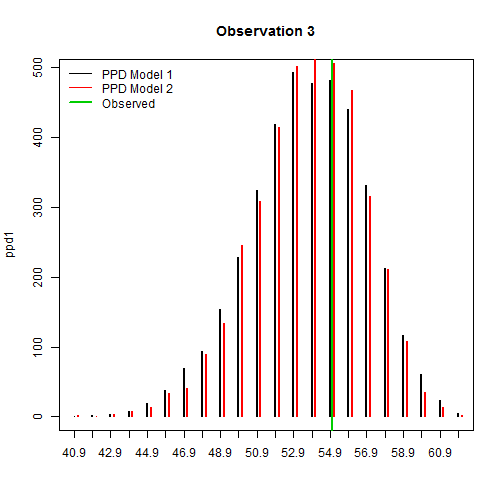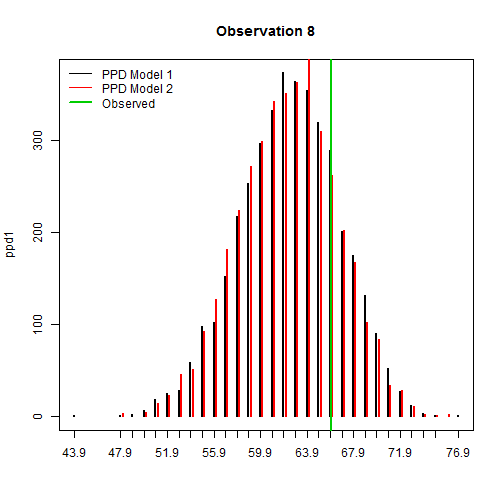## Summarize results

  y     <- cbind(Y,Y) # Make data the same size/format as predictions
BIAS  <- colMeans(Y_mean-y)
MSE   <- colMeans((Y_mean-y)^2)
COV   <- colMeans( (Y_low <= y) & (y <= Y_high))
WIDTH <- colMeans(Y_high-Y_low)

plot(Y,Y_mean[,1],pch=19,
xlim=c(0,100),ylim=c(0,100),
xlab="Observed",ylab="Predicted")
points(Y,Y_mean[,2],col=2,pch=19)
abline(0,1)
legend("topleft",c("Model 1","Model 2"),pch=19,col=1:2,bty="n")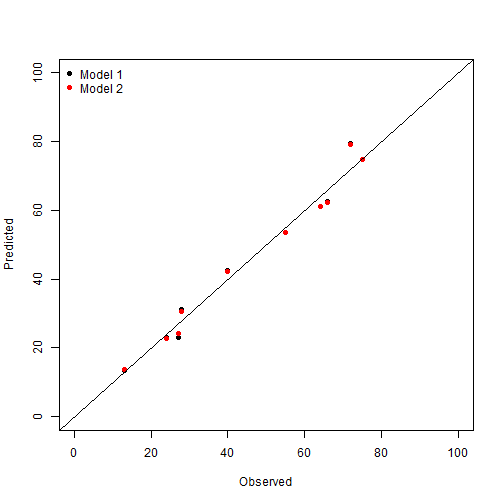OUT   <- cbind(BIAS,MSE,MAD,COV,WIDTH)
OUT   <- round(OUT,2)
kable(OUT)